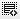## npv and IRR0
what is more important,NPV or IRR??0

DEFINITION of 'Net Present Value - NPV'
The difference between the present value of cash inflows and the present value of cash outflows. NPV is used in capital budgeting to analyze the profitability of a projected investment or project.

A positive net present value indicates that the projected earnings generated by a project or investment (in present dollars) exceeds the anticipated costs (also in present dollars). Generally, an investment with a positive NPV will be a profitable one and one with a negative NPV will result in a net loss. This concept is the basis for the Net Present Value Rule, which dictates that the only investments that should be made are those with positive NPV values.
The average accounting return method of evaluating business investments is based on using the accounting rate of return for a specified number of years to arrive at an average rate of return for the period. Typically, the average accounting return method is used as a tool for comparing potential profitability of two or more investment opportunities
Net Present value is more important0

he difference between the present value of cash inflows and the present value of cash outflows. NPV is used in capital budgeting to analyze the profitability of a projected investment or project.

A positive net present value indicates that the projected earnings generated by a project or investment (in present dollars) exceeds the anticipated costs (also in present dollars). Generally, an investment with a positive NPV will be a profitable one and one with a negative NPV will result in a net loss. This concept is the basis for the Net Present Value Rule, which dictates that the only investments that should be made are those with positive NPV values.
The average accounting return method of evaluating business investments is based on using the accounting rate of return for a specified number of years to arrive at an average rate of return for the period. Typically, the average accounting return method is used as a tool for comparing potential profitability of two or more investment opportunities
Net Present value is more important0

NPV and IRR are two approaches used in capital budgeting decisions to evaluate given projects .All other things being equal, using internal rate of return (IRR) and net present value (NPV) measurements to evaluate projects often results in the same findings. However, there are a number of projects for which using IRR is not as effective as using NPV to discount cash flows. IRR's major limitation is also its greatest strength: it uses one single discount rate to evaluate every investment.

Although using one discount rate simplifies matters, there are a number of situations that cause problems for IRR. If an analyst is evaluating two projects, both of which share a common discount rate, predictable cash flows, equal risk, and a shorter time horizon, IRR will probably work. The catch is that discount rates usually change substantially over time. For example, think about using the rate of return on a T-bill in the last 20 years as a discount rate. One-year T-bills returned between 1% and 12% in the last 20 years, so clearly the discount rate is changing.

Click on thisicon to add code snippet.